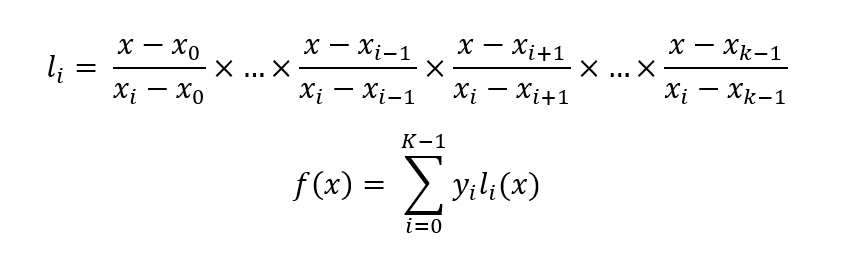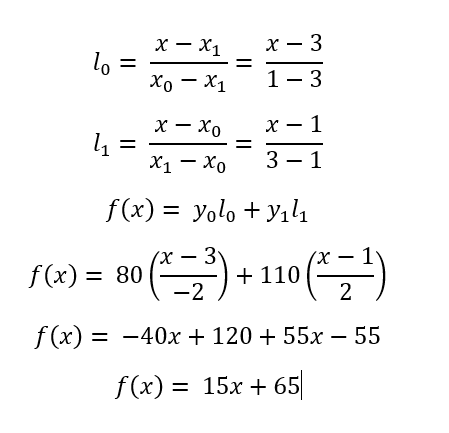# Shamir-s-Secret-Sharing-Algorithm-Cryptography

Shamir’s Secret Sharing Algorithm: Shamir’s Secret Sharing is an algorithm in cryptography created by Adi Shamir. The main aim of this algorithm is to divide secret that needs to be encrypted into various unique parts.

## Introduction

Cryptography is a technique of securing information and communications through the use of codes so that only those person for whom the information is intended can understand it and process it. Thus preventing unauthorized access to information. The prefix “crypt” means “hidden” and suffix graphy means “writing”. In this article, a type of cryptographic technique, Shamir’s secret sharing algorithm is discussed.

## Getting started

• Let’s say S is the secret that we wish to encode.
• It is divided into N parts: S1, S2, S3, …., Sn.
• After dividing it, a number K is chosen by the user in order to decrypt the parts and find the original secret.
• It is chosen in such a way that if we know less than K parts, then we will not be able to find the secret S (i.e.) the secret S can not be reconstructed with (K – 1) parts or fewer.
• If we know K or more parts from S1, S2, S3, …., Sn, then we can compute/reconstructed our secret code S easily. This is conventionally called (K, N) threshold scheme.

Approach: The main idea behind the Shamir’s Secret Sharing Algorithm lies behind the concept that for the given K points we can find a polynomial equation with the degree (K – 1).

### Example

• For the given two points, (x1, y1) and (x2, y2) we can find a linear polynomial ax + by = c.
• Similarly, for the given three points, we can find a quadratic polynomial ax2 + bx + cy = d.
• So, the idea is to build a polynomial with the degree (K – 1) such that the constant term is the secret code and the remaining numbers are random and this constant term can be found by using any K points out of N points generated from this polynomial by using Legrange’s Basis Polynomial.

Let the secret code S = 65, N = 4, K = 2.

Initially, in order to encrypt the secret code, we build a polynomial of degree (K – 1).
Therefore, let the polynomial be y = a + bx. Here, the constant part ‘a’ is our secret code.
Let b be any random number, say b = 15.
Therefore, for this polynomial y = 65 + 15x, we generate N = 4 points from it.
Let those 4 points be (1, 80), (2, 95), (3, 110), (4, 125). Clearly, we can generate the initial polynomial from any two of these 4 points and in the resulting polynomial, the constant term a is the required secret code.
In order to reconstruct the given polynomial back, the Lagrange basis Polynomial is used.
The main concept behind the Lagrange polynomial is to form the Lagrange’s identities first and the summation of these identities give us the required function which we need to find from the given points. The following equations show how to compute them:For example, let’s say we wish to compute the given equation by using K = 2 points from the selected four points- (1, 80), (3, 110). The following illustration shows the step by step solution for this:### Expected output

``````1 602
2 1139
3 1676
4 2213
We can generate Secret from any of 2 Parts
Our Secret Code is : 65
``````

The implimentation of this can be found in the ` SSSA.cpp` file.

Thankyou!!!

View Github